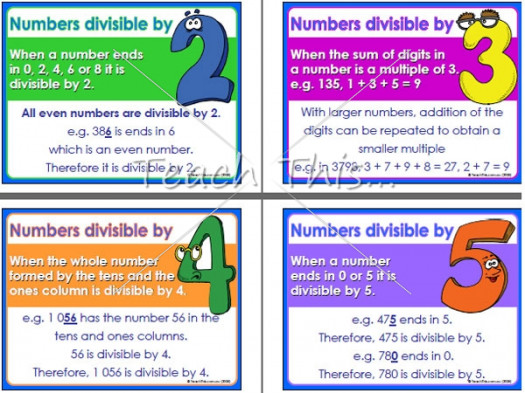10 Questions | Attempts: 120
ShareSettingsIn mathematics, an equation is a statement of an equality containing one or more variables. Solving the equation consists of determining which values of the variables make the equality true. So what do you know about one-step addition and subtraction equations?Back to top